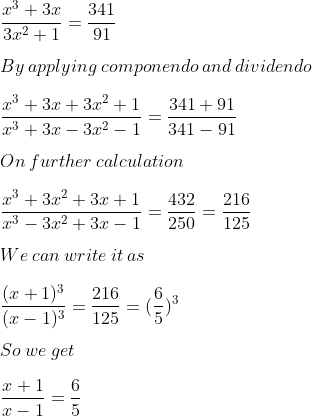Guru

# Using the properties of proportion, solve the following equation for x; given x³+3x/3x²+1=341/91

• 0

This is an important ques from the Book ML Aggarwal class 10th,, chapter – 7, ratio and proportion.

We have been given the equation of variable x and we have to find the value of x using properties of proportion.

Question 18, 7.3

Share

1. Solution:

It is given thatBy cross multiplication

6x – 6 = 5x + 5

6x – 5x = 5 + 6

x = 11

• 2